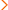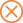Home > ed > SAT Math - An overview of the SAT Math sections

# SAT Math - An overview of the SAT Math sections

The SAT Math sections consist of a total of 54 questions: 44 multiple-choice questions and 10 student-produced response questions. The five-choice multiple-choice questions require students to solve a problem and select the best answer from the available choices. The student-produced response questions require students to solve a problem and grid the correct answer; the questions do not provide answer choices.

The SAT Math questions draw from four areas of math: number and operations; algebra and functions; geometry and measurement; and data analysis, statistics, and probability. Below you can find more detailed information about the specific skills these questions test. Please note that the information below is for the current version of the SAT that will be administered through January of 2016. For information on the new SAT that will be administered beginning in March 2016, visit our page with information on Navigating the New SAT.

Math Area Examples # of Questions

Number and Operations

properties of integers; arithmetic word problems; number lines; squares and square roots; fractions and rational numbers; factors, multiples, and remainders; prime numbers; ratios, proportions, and percents; sequences; sets; counting problems; and logical reasoning

11-13 questions

Algebra and Functions

operations on algebraic expressions; factoring; exponents; evaluating expressions with exponents and roots; solving equations; absolute value; direct translation into mathematical expressions; inequalities; systems of linear equations and inequalities; factoring; rational equations and inequalities; direct and inverse variation; word problems; and functions

19-21 questions

Geometry and Measurement

geometric notation; points and lines; angles in the plane; triangles; quadrilaterals; areas and perimeters; other polygons; circles; solid geometry; geometric perception; coordinate geometry; and transformations

14-16 questions

Data Analysis, Statistics, and Probability

data interpretation; statistics; elementary probability; and geometric probability

6-7 questions

To access sample SAT Math multiple-choice questions, visit the College Board website.

To access sample SAT Math student-produced response questions, visit the College Board website.

Related Topics

LEARN MORE